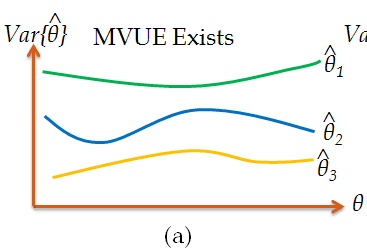Get inspired by the success stories of our students in IIT JAM MS, ISI  MStat, CMI MSc Data Science.  Learn More

# ISI MStat PSB 2004 Problem 6 | Minimum Variance Unbiased EstimatorsThis is a very beautiful sample problem from ISI MStat PSB 2004 Problem 6. It's a very simple problem, and its simplicity is its beauty . Fun to think, go for it !!

## Problem- ISI MStat PSB 2004 Problem 6

Let $Y_1,Y_2.Y_3$, and $Y_4$ be four uncorrelated random variables with

$E(Y_i) =i\theta ,$ $Var(Y_i)= i^2 {\sigma}^2,$ , $i=1,2,3,4$ ,

where $\theta$ and $\sigma$ (>0) are unknown parameters. Find the values of $c_1,c_2,c_3,$ and $c_4$ for which $\sum_{i=1}^4{c_i Y_i}$ is unbiased for $\theta$ and has least variance.

### Prerequisites

Unbiased estimators

Minimum-Variance estimators

Cauchy-Schwarz inequality

## Solution :

This is a very simple and cute problem, just do as it is said...

for , $\sum_{i=1}^4{c_i Y_i}$ to be an unbiased estimator for $\theta$ , then it must satisfy,

$E(\sum_{i=1}^4{c_i Y_i} )= \theta \Rightarrow \sum_{i=1}^4{c_i E(Y_i)}= \theta \Rightarrow \sum_{i=1}^4{c_i i \theta} = \theta$

so, $\sum_{i=1}^4 {ic_i}=1 .$ ......................(1)

So, we have to find $c_1,c_2,c_3,$ and $c_4$, such that (1), is satisfied . But hold on there is some other conditions also.

Again, since the given estimator will also have to be minimum variance, lets calculate the variance of $\sum_{i=1}^4{c_i Y_i}$ ,

$Var(\sum_{i=1}^4{c_i Y_i})= \sum_{i=1}^4{c_i}^2Var( Y_i)=\sum_{i=1}^4{i^2 {c_i}^2 {\sigma}^2 }.$...............................................(2)

So, for minimum variance, $\sum_{i=1}^4{i^2{c_i}^2 }$ must be minimum in (2).

So, we must find $c_1,c_2,c_3,$ and $c_4$, such that (1), is satisfied and $\sum_{i=1}^4{i^2{c_i}^2 }$ in (2) is minimum.

so, minimizing $\sum_{i=1}^4{i^2{c_i}^2 }$ when it is given that $\sum_{i=1}^4 {ic_i}=1$ ,

What do you think, what should be our technique of minimizing $\sum_{i=1}^4{i^2{c_i}^2 }$ ???

For, me the beauty of the problem is hidden in this part of minimizing the variance. Can't we think of Cauchy-Schwarz inequality to find the minimum of, $\sum_{i=1}^4{i^2{c_i}^2 }$ ??

So, using CS- inequality, we have,

$(\sum_{i=1}^4{ic_i})^2 \le n \sum_{i=1}^4{i^2{c_i}^2} \Rightarrow \sum_{i=1}^4 {i^2{c_i}^2} \ge \frac{1}{n}.$ ...........(3). [ since $\sum_{i=1}^4 {ic_i}=1$ ].

now since $\sum_{i=1}^4{i^2{c_i}^2 }$ is minimum the equality in (3) holds, i.e. $\sum_{i=1}^4{i^2{c_i}^2 }=\frac{1}{n}$ .

and we know the equality condition of CS- inequality is, $\frac{1c_1}{1}=\frac{2c_2}{1}=\frac{3c_3}{1}=\frac{4c_4}{1}=k$ (say),

then $c_i= \frac{k}{i}$ for i=1,2,3,4 , where k is some constant .

Again since, $\sum_{i=1}^4{ic_i} =1 \Rightarrow 4k=1 \Rightarrow k= \frac{1}{4}$ . Hence the solution concludes .

## Food For Thought

Let's, deal with some more inequalities and behave Normal !

Using, Chebyshev's inequality we can find a trivial upper bound for $P(|Z| \ge t)$, where $Z \sim n(0,1)$ and t>0 ( really !! what's the bound ?). But what about some non-trivial bounds, sharper ones perhaps !! Can you show the following,

$\sqrt{\frac{2}{\pi}}\frac{t}{1+t^2}e^{-\frac{t^2}{2}} \le P(|Z|\ge t) \le \sqrt{\frac{2}{\pi}}\frac{e^{-\frac{t^2}{2}}}{t}$ for all t>0.

also, verify this upper bound is sharper than the trivial upper bound that one can obtain.

## Subscribe to Cheenta at Youtube

This is a very beautiful sample problem from ISI MStat PSB 2004 Problem 6. It's a very simple problem, and its simplicity is its beauty . Fun to think, go for it !!

## Problem- ISI MStat PSB 2004 Problem 6

Let $Y_1,Y_2.Y_3$, and $Y_4$ be four uncorrelated random variables with

$E(Y_i) =i\theta ,$ $Var(Y_i)= i^2 {\sigma}^2,$ , $i=1,2,3,4$ ,

where $\theta$ and $\sigma$ (>0) are unknown parameters. Find the values of $c_1,c_2,c_3,$ and $c_4$ for which $\sum_{i=1}^4{c_i Y_i}$ is unbiased for $\theta$ and has least variance.

### Prerequisites

Unbiased estimators

Minimum-Variance estimators

Cauchy-Schwarz inequality

## Solution :

This is a very simple and cute problem, just do as it is said...

for , $\sum_{i=1}^4{c_i Y_i}$ to be an unbiased estimator for $\theta$ , then it must satisfy,

$E(\sum_{i=1}^4{c_i Y_i} )= \theta \Rightarrow \sum_{i=1}^4{c_i E(Y_i)}= \theta \Rightarrow \sum_{i=1}^4{c_i i \theta} = \theta$

so, $\sum_{i=1}^4 {ic_i}=1 .$ ......................(1)

So, we have to find $c_1,c_2,c_3,$ and $c_4$, such that (1), is satisfied . But hold on there is some other conditions also.

Again, since the given estimator will also have to be minimum variance, lets calculate the variance of $\sum_{i=1}^4{c_i Y_i}$ ,

$Var(\sum_{i=1}^4{c_i Y_i})= \sum_{i=1}^4{c_i}^2Var( Y_i)=\sum_{i=1}^4{i^2 {c_i}^2 {\sigma}^2 }.$...............................................(2)

So, for minimum variance, $\sum_{i=1}^4{i^2{c_i}^2 }$ must be minimum in (2).

So, we must find $c_1,c_2,c_3,$ and $c_4$, such that (1), is satisfied and $\sum_{i=1}^4{i^2{c_i}^2 }$ in (2) is minimum.

so, minimizing $\sum_{i=1}^4{i^2{c_i}^2 }$ when it is given that $\sum_{i=1}^4 {ic_i}=1$ ,

What do you think, what should be our technique of minimizing $\sum_{i=1}^4{i^2{c_i}^2 }$ ???

For, me the beauty of the problem is hidden in this part of minimizing the variance. Can't we think of Cauchy-Schwarz inequality to find the minimum of, $\sum_{i=1}^4{i^2{c_i}^2 }$ ??

So, using CS- inequality, we have,

$(\sum_{i=1}^4{ic_i})^2 \le n \sum_{i=1}^4{i^2{c_i}^2} \Rightarrow \sum_{i=1}^4 {i^2{c_i}^2} \ge \frac{1}{n}.$ ...........(3). [ since $\sum_{i=1}^4 {ic_i}=1$ ].

now since $\sum_{i=1}^4{i^2{c_i}^2 }$ is minimum the equality in (3) holds, i.e. $\sum_{i=1}^4{i^2{c_i}^2 }=\frac{1}{n}$ .

and we know the equality condition of CS- inequality is, $\frac{1c_1}{1}=\frac{2c_2}{1}=\frac{3c_3}{1}=\frac{4c_4}{1}=k$ (say),

then $c_i= \frac{k}{i}$ for i=1,2,3,4 , where k is some constant .

Again since, $\sum_{i=1}^4{ic_i} =1 \Rightarrow 4k=1 \Rightarrow k= \frac{1}{4}$ . Hence the solution concludes .

## Food For Thought

Let's, deal with some more inequalities and behave Normal !

Using, Chebyshev's inequality we can find a trivial upper bound for $P(|Z| \ge t)$, where $Z \sim n(0,1)$ and t>0 ( really !! what's the bound ?). But what about some non-trivial bounds, sharper ones perhaps !! Can you show the following,

$\sqrt{\frac{2}{\pi}}\frac{t}{1+t^2}e^{-\frac{t^2}{2}} \le P(|Z|\ge t) \le \sqrt{\frac{2}{\pi}}\frac{e^{-\frac{t^2}{2}}}{t}$ for all t>0.

also, verify this upper bound is sharper than the trivial upper bound that one can obtain.

## Subscribe to Cheenta at Youtube

This site uses Akismet to reduce spam. Learn how your comment data is processed.

### One comment on “ISI MStat PSB 2004 Problem 6 | Minimum Variance Unbiased Estimators”

1.abhishek sinha says:

In the final step, k must be equal to 1/4 (due to unbiasedness).

### Knowledge Partner

Cheenta is a knowledge partner of Aditya Birla Education Academy